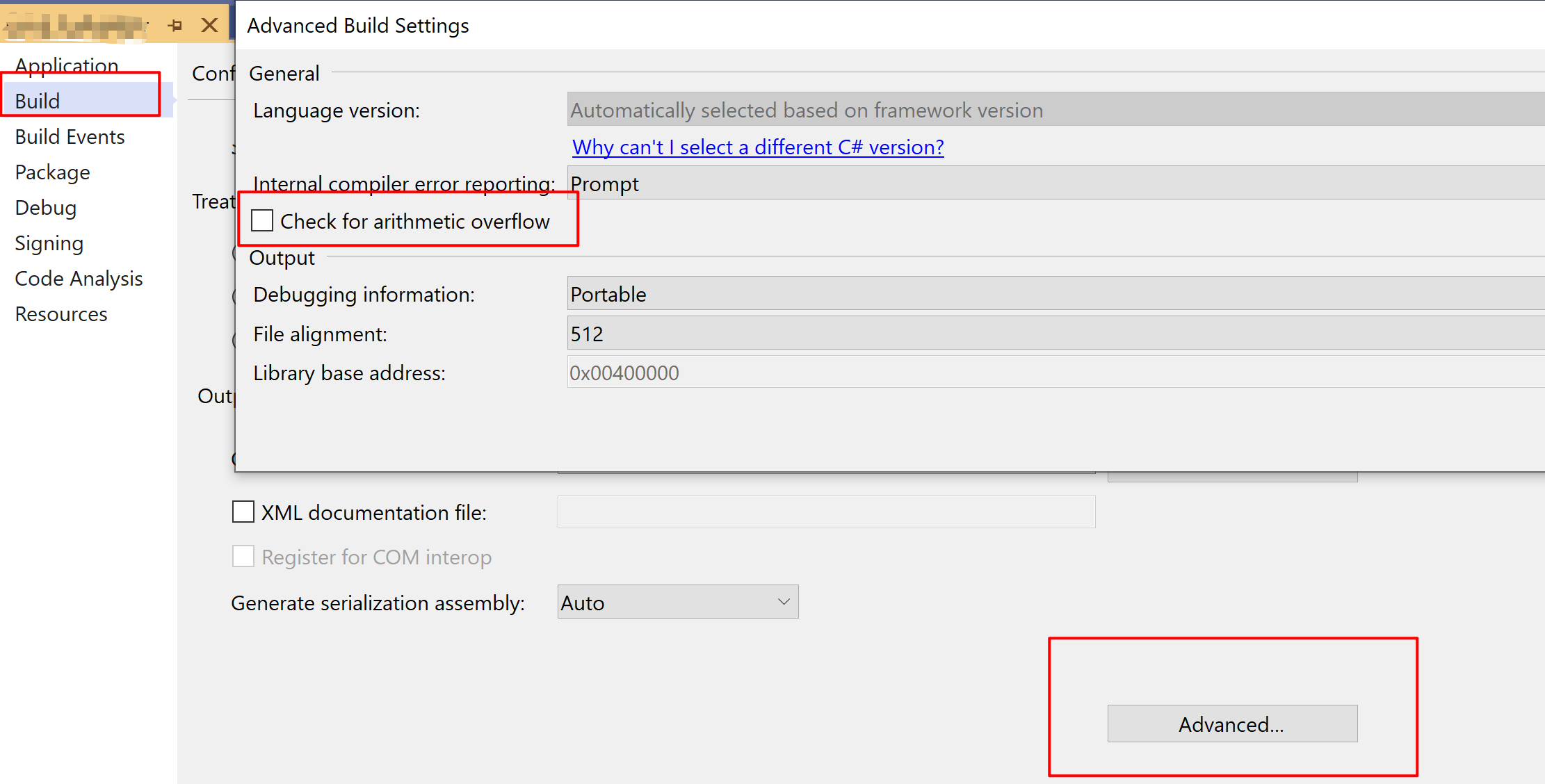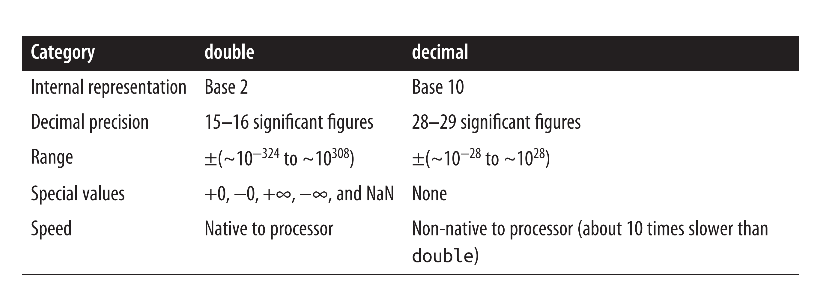# Luna Tech

Tutorials For Dummies.

# 0. 回顾：整数型

short, ushort, byte, sbyte, int, uint, long, ulong

# 1. Arithmetic Operators 运算符

+ - * / %

% 是余数，/ 是除法

## 除法 Division

10/3 = 3

10%3 = 1

10/0 => DivideByZeroException, compile-time error

## Overflow

Console.WriteLine(int.MinValue); // -2^31 = -2147483648
Console.WriteLine(int.MaxValue); // 2^31 -1 = 2147483647
int a = int.MinValue;
a--; // a = -2^31 - 1
Console.WriteLine(a); // 2147483647
Console.WriteLine(a == int.MaxValue); // True


int x = int.MaxValue + 1; // compile time error


### 如何捕获溢出异常呢？

checked 对++，–，+，-，*，/，以及整数型之间的 explicit conversion 都是有效的。

checked 会增加一些 performance cost，但属于可接受范围。

int a = 1000000;
int b = 1000000;

// 只 check 这行代码里的表达式
int c = checked (a * b);

// check block 里面的所有表达式
checked {
c = a * b;
}


int x = int.MaxValue;
int y1 = unchecked (x + 1);
int y2 = unchecked (int.MaxValue + 1); // no compile-time error
unchecked {int z = x + 1}# 2. Increment and Decrement Operators 增减符

++ --

• i++（后++）是先用后算，先把 i 的值用来做比较，再把 i 的值 + 1；
• ++i（前++）是先算后用，先把 i 的值 + 1，再使用这个新的值作比较；

int x = 0, y = 0;
Console.WriteLine(x++); // Output: 0, x = 1
Console.WriteLine(++y); // Output: 1, y = 1


# 3. 8-bit 和 16-bit 的整数型 (byte, short)

short a = 1, b = 1;
// compile-time error
// a and b are implicitly converted to int
short c = a + b;
short d = (short)(a + b); // no error


# 4. 特殊的 float 和 double value

Console.WriteLine(double.NegativeInfinity); // -∞
Console.WriteLine(double.PositiveInfinity); // ∞
Console.WriteLine(double.NaN); // NaN, Not a Number
Console.WriteLine(-0.0); // -0

Console.WriteLine(float.NegativeInfinity); // -∞
Console.WriteLine(float.PositiveInfinity); // ∞
Console.WriteLine(float.NaN); // NaN
Console.WriteLine(-0.0f); // -0


## 特殊值之间的运算

1. NaN 永远不可能等于另一个值，即使是 NaN 自己，判定 NaN 的方法是 IsNaN 或者 object.Equals；
2. NaN 可以用来代表程序的一个初始化状态；
Console.WriteLine( 1.0 / 0.0 ); // ∞
Console.WriteLine(-1.0 / -0.0); // ∞
Console.WriteLine(-1.0 / 0.0 ); // -∞
Console.WriteLine( 1.0 / -0.0); // -∞

Console.WriteLine( 0.0 / 0.0); // NaN
Console.WriteLine( (1.0 / 0.0) - (1.0 / 0.0)); // NaN

Console.WriteLine( 0.0 / 0.0 == 0.0 / 0.0); // False
Console.WriteLine(double.IsNaN(0.0 / 0.0)); // True
Console.WriteLine(object.Equals((0.0 / 0.0), (0.0 / 0.0))); // True
Console.WriteLine(object.Equals((0.0 / 0.0), double.NaN); // True


float and double follow the specification of the IEEE 754 format types, supported natively by almost all processors. You can find detailed information on the behavior of these types on the IEEE website.

# 5. double VS decimal

• double 用于科学计算
• decimal 用于金融计算和人为制造的数值计算double 和 float 内部是以二进制表示的，所以只有二进制的数字才能被精确表示，而大部分 10 进制的数字都不能被精确表示。

double 和 decimal 都不能精确表示除不尽的分数（fractional number）。

decimal m = 1M / 6M; // 0.1666666666666666666666666667
double d = 1.0 / 6.0; // 0.16666666666666666

Console.WriteLine(m + m + m + m + m + m == 1M); // False
Console.WriteLine(d + d + d + d + d + d == 1.0); // False

Console.WriteLine(m * 6 == 1M); // False
Console.WriteLine(d * 6 == 1.0); // True